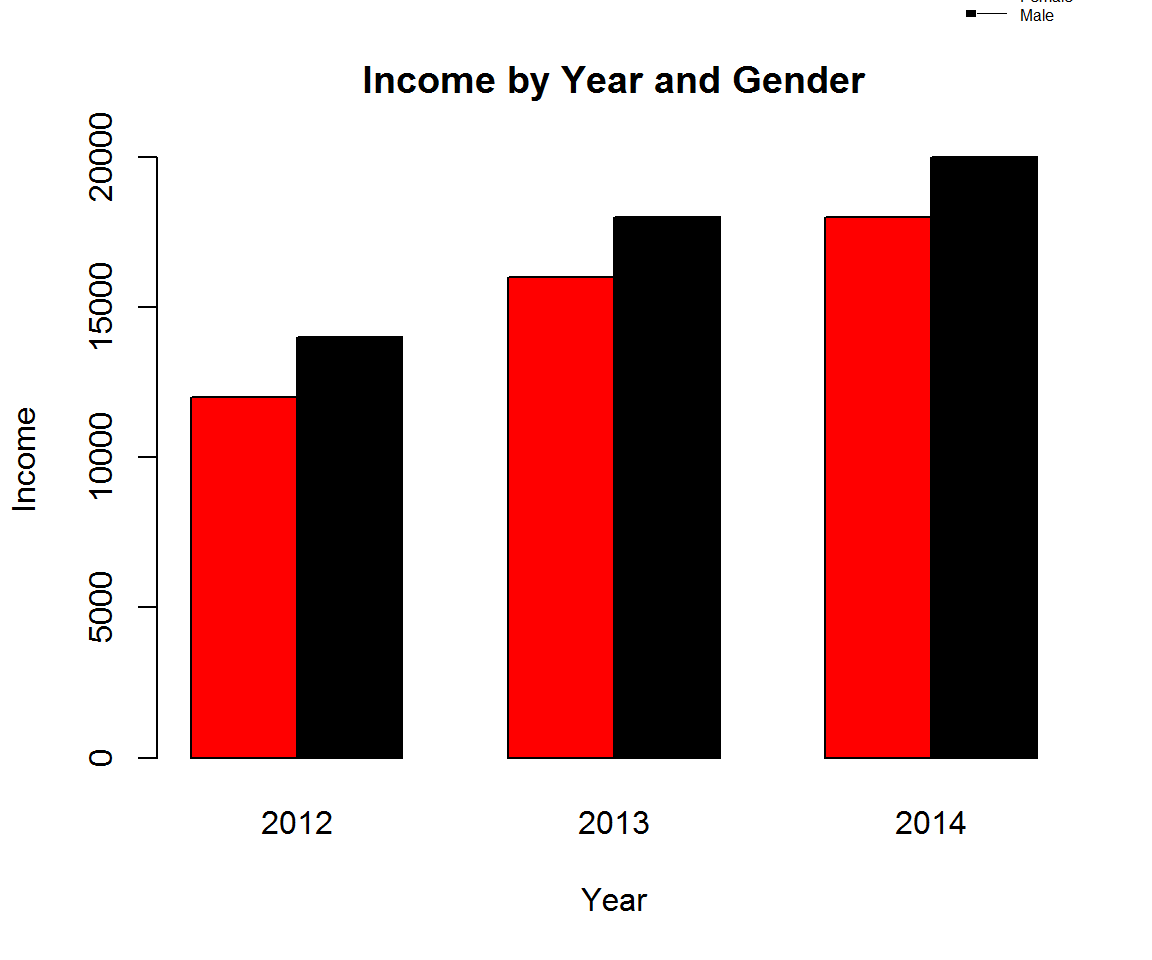# Clustered Column Chart using base R Graphic

Column chart is used to compared numeric values across categories. Normally when categorical values are in cronological or sequential order, we use column chart. When we want users to compare values across categories, we should use Bar Chart/horizontal bars.

Type of Column Charts

• Normal Column Chart
• Grouped or Clustered Column Chart
• Stacked Column Chart and 100% Stacked Column Chart

Example of different Column Charts Types. In this blog, the focus will be more on Grouped Column Chart.

par(mfrow=c(1,3))
# data frame for simple column chart
col <- data.frame(sno=c(1,2,3),inc=c(12000,20000,27000))
# plot simple column chart
barplot(height=col\$inc,
main="Simple Column Chart",
names.arg = c(2012,2013,2014) )
# data for clustered column chart
clus.col <- data.frame(inc2013=c(12000,20000,27000),
inc2014=c(14000,22000,22000))
clus.col1 <- do.call(rbind, clus.col) # transform data
# Clustered Column Chart
barplot(clus.col1 ,beside = TRUE,
main="Grouped Column Chart",
legend.text = c("Female","Male"),
names.arg = c(2012,2013,2014)
)
## Data for Stacked Column Chart
Income2013 <- c(12000,14000)
Income2014 <- c(22000,23000)
Gender <- c("Female","Male")
stack.col <- data.frame(Gender,Income2013,Income2014)
barplot(as.matrix(stack.col[,2:3] ),
main="Stacked Column Chart",
legend.text = stack.col[,1],
names.arg = c("2013","2014")
)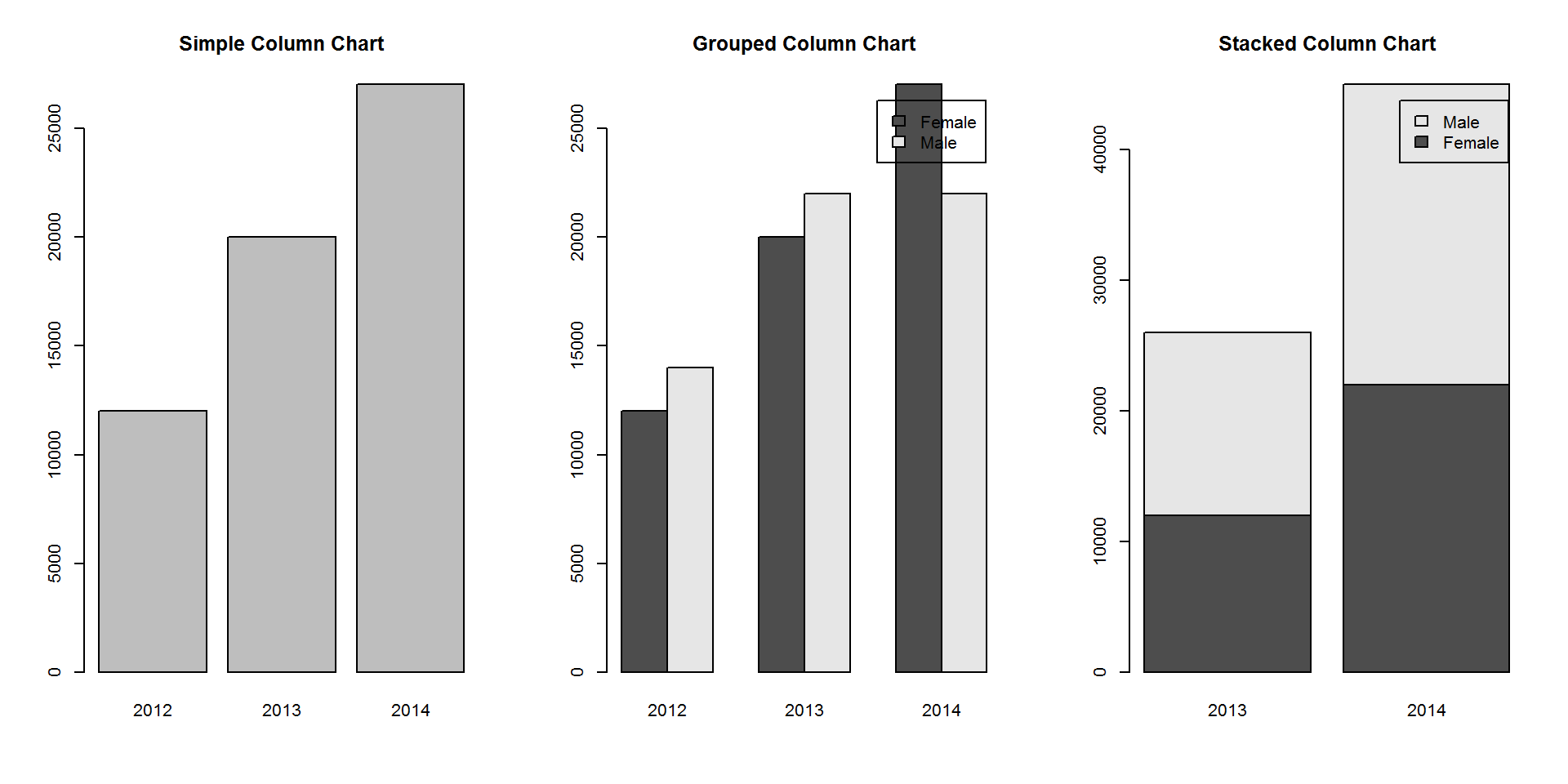For normal column chart using base R graphic, http://dni-institute.in/blogs/visualization-in-r-cute-column-chart-part-1/

#### Getting started with Clustered or Grouped Column Chart

One of the key steps in getting chart right is to prepare data in the relevant format.

In a grouped column chart, have two categorical dimensions and a numeric vector indicating height of the columns.

But the data should be in a transformed form. First column for category presenting sequence of columns (legend) and then a list vectors for providing heights. The number of vectors will show as different groups of columns.

Also, one of the steps to provide the input data in a matrix format.

par(mfrow=c(1,1))
# data for clustered column chart
clus.col <- data.frame(Gender=c("Female","Male"),
inc.2012 = c(12000,14000),
inc.2013 = c(16000,18000),
inc.2014 = c(18000,20000)
)
# Clustered Column Chart
barplot(as.matrix(clus.col[,2:4]) ,
beside = TRUE,
main="Grouped Column Chart",
legend.text = c("Female","Male"),
names.arg = c(2012,2013,2014)
)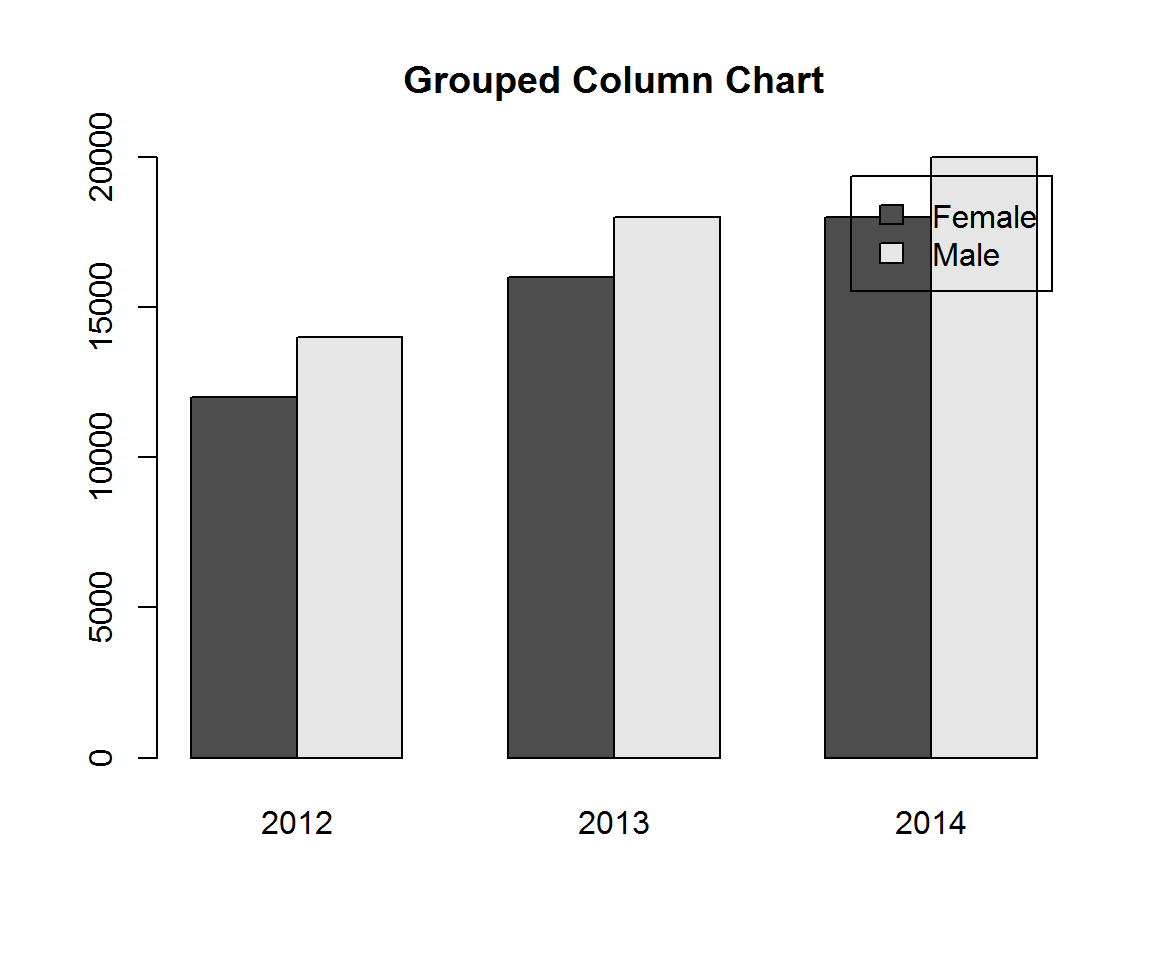#### Improve readability of the chart.

Provide axis lables to improve readability of the chart. xlab and ylab can be used to give label to the axis x and y respectively.

# Clustered Column Chart
barplot(as.matrix(clus.col[,2:4]) ,
beside = TRUE,
main="Income by Year and Gender",
xlab="Year",
ylab="Income",
legend.text = c("Female","Male"),
names.arg = c(2012,2013,2014)
)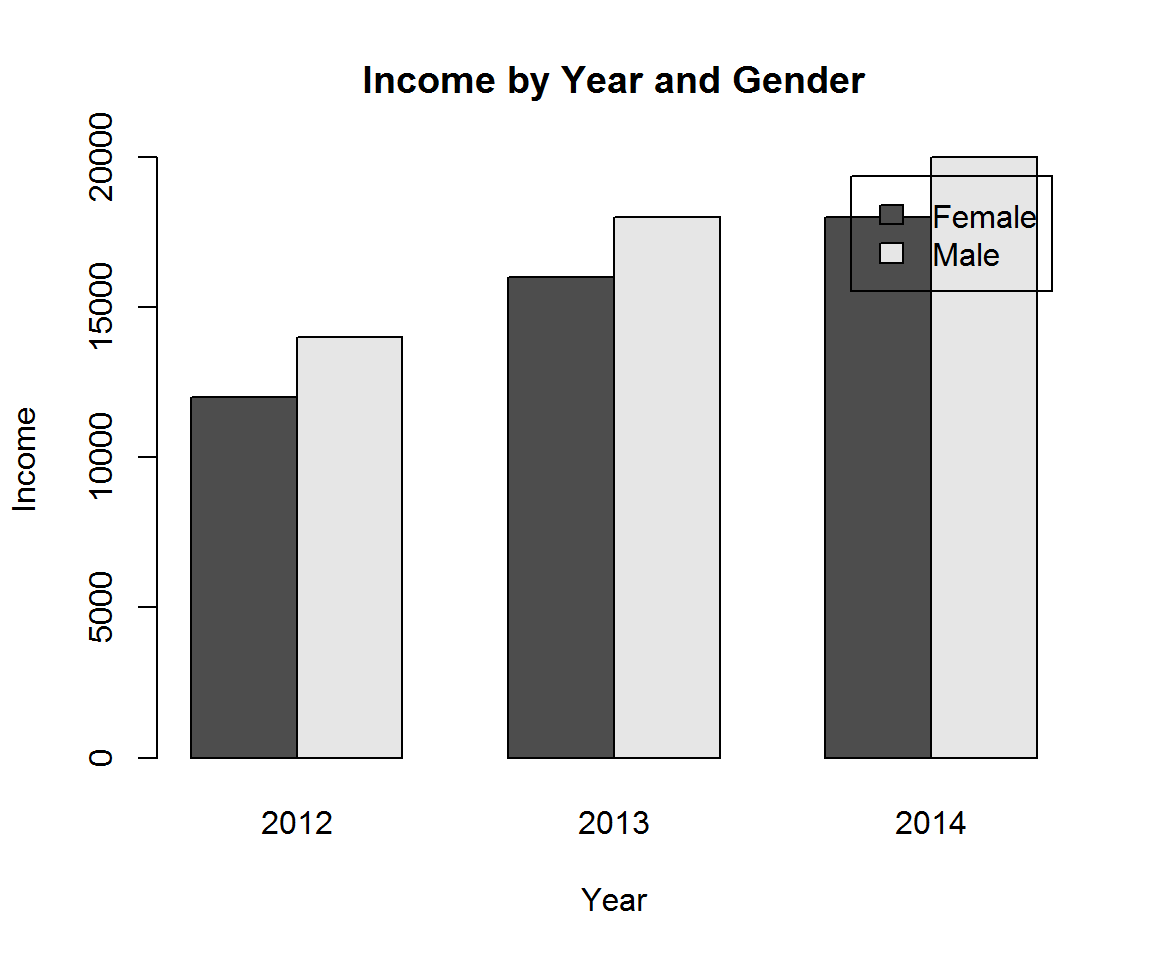##### Change color of the bars or columns.
# Clustered Column Chart
barplot(as.matrix(clus.col[,2:4]) ,
beside = TRUE,
main="Income by Year and Gender",
xlab="Year",
ylab="Income",
legend.text = c("Female","Male"),
names.arg = c(2012,2013,2014),
col=c("red","black")
)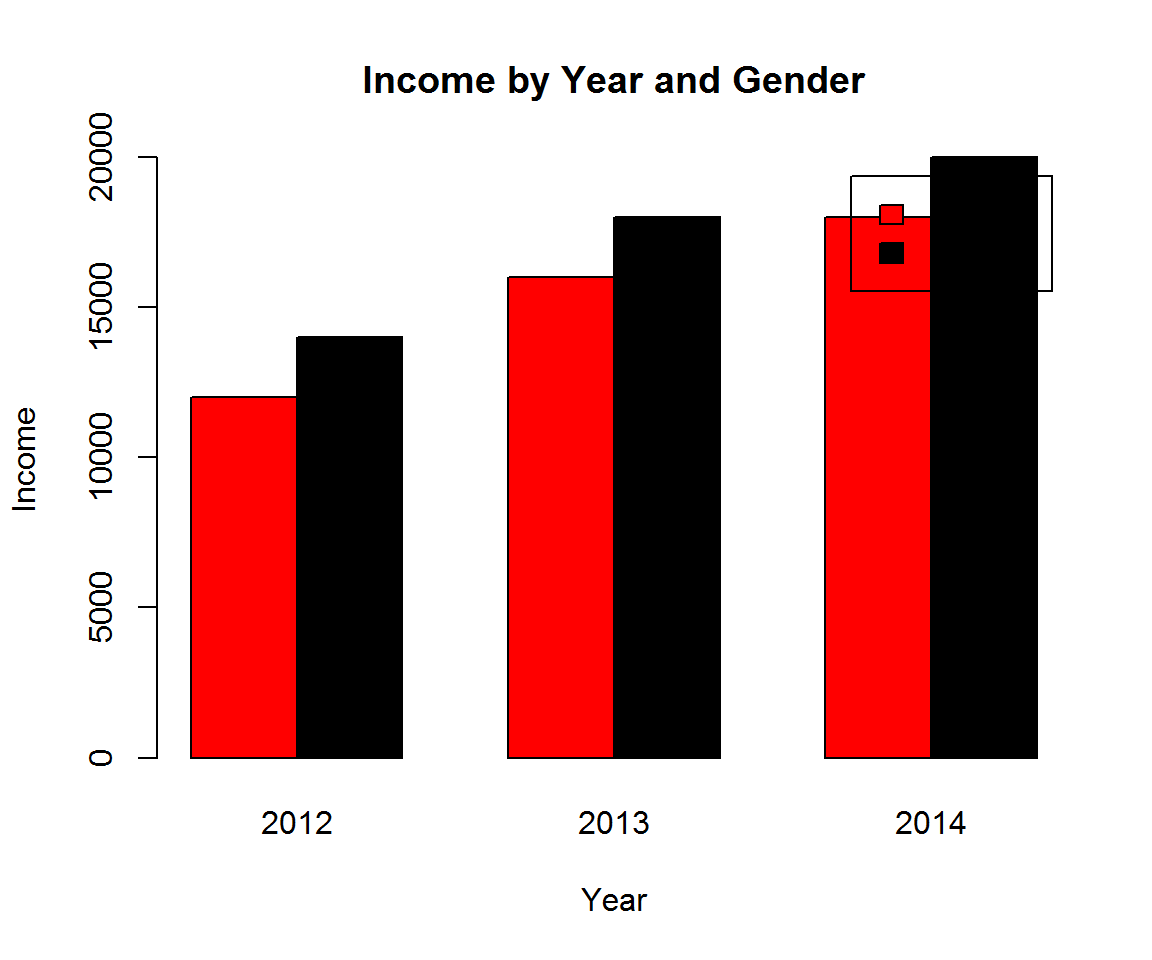##### Formatting of Legend

legend function provides a number of options to format legend.

# Clustered Column Chart
barplot(as.matrix(clus.col[,2:4]) ,
beside = TRUE,
main="Income by Year and Gender",
xlab="Year",
ylab="Income",
names.arg = c(2012,2013,2014),
col=c("red","black")
)
par(xpd=TRUE) ## allow plotting to figure region instead of just plot region
legend( "topright",  ## position of the legend
inset=c(-0.01,-.3), ## allow tweaking of the legend position
legend=c("Female","Male"), ## legends
border="white", ## legend box line
fill=c("red","black"), ## legend colors
lwd=0, ## line width around legend box
box.col="white"  , ## legend box
cex =0.5,  ## size of the legend text and box
bg=NULL  ## legend box color, NULL indicate transparent
)